# Darin Kapanjie's Videos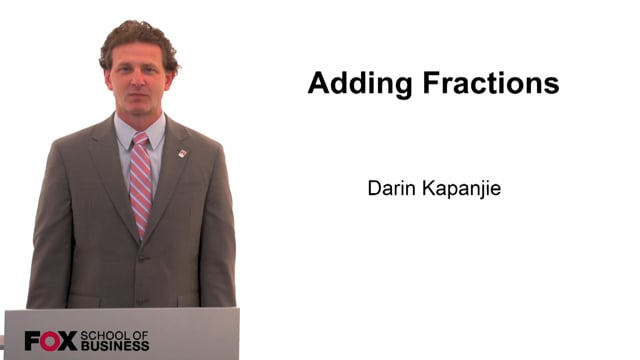Total Running Time: 08:01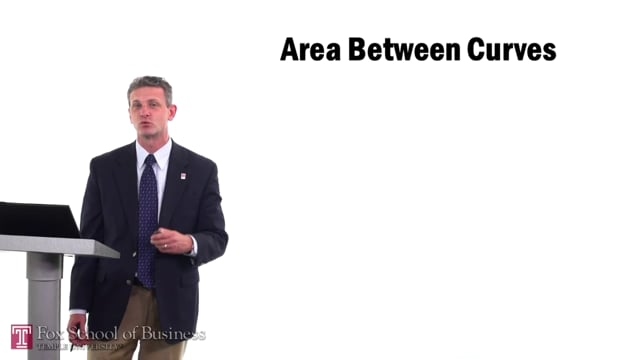Area Between Curves
Total Running Time: 17:51
Area Between Curves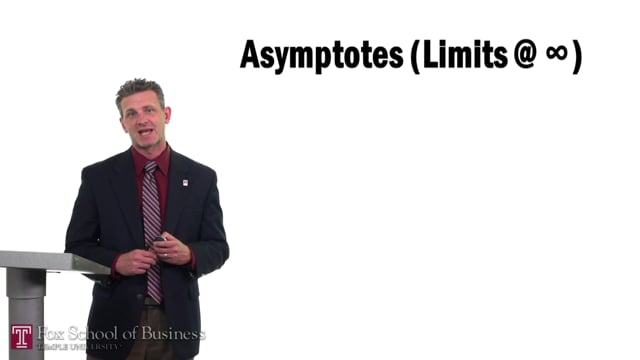Asymptotes
Total Running Time: 13:45
AsymptotesChain Rule
Total Running Time: 14:50
Chain RuleCorrelation
Total Running Time: 08:56
CorrelationData
Total Running Time: 08:38
DataElasticity of Demand
Total Running Time: 18:27
Elasticity of DemandEquations of Lines
Total Running Time: 22:59
Equations of LinesExpected Value
Total Running Time: 13:22
Expected ValueExponential Functions
Total Running Time: 15:50
Exponential Functions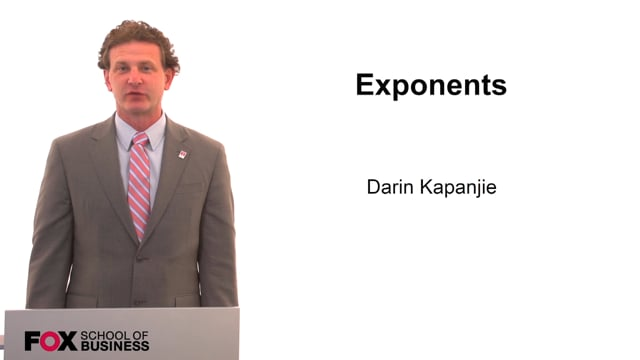Exponents
Total Running Time: 09:19
ExponentsFactoring
Total Running Time: 22:52
Factoring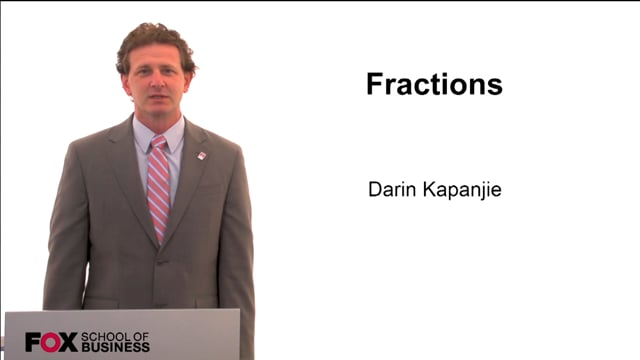Fractions
Total Running Time: 07:31
FractionsFunctions
Total Running Time: 29:35
FunctionsFunctions and Models
Total Running Time: 12:43
Functions and ModelsGraphs
Total Running Time: 22:06
GraphsInterval Notation
Total Running Time: 05:07
Interval Notation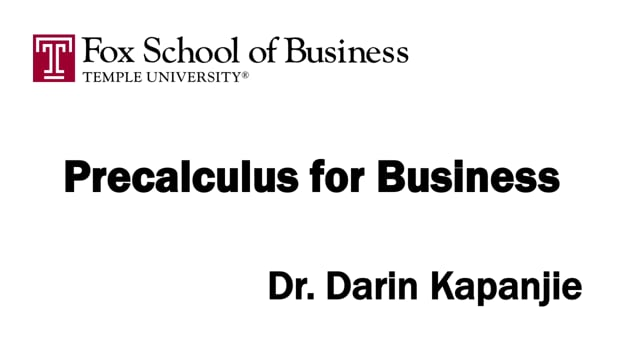Introduction
Total Running Time: 01:58
IntroductionLinear Inequalities
Total Running Time: 17:55
Linear InequalitiesLinear Regression
Total Running Time: 16:09
Linear RegressionLogarithmic Functions
Total Running Time: 15:19
Logarithmic FunctionsManipulating Formulas
Total Running Time: 10:37
Manipulating FormulasMarginal Functions
Total Running Time: 08:00
Marginal Functions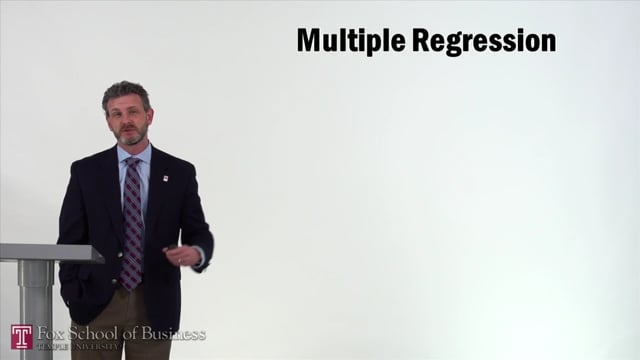Multiple Regression
Total Running Time: 16:37
Multiple Regression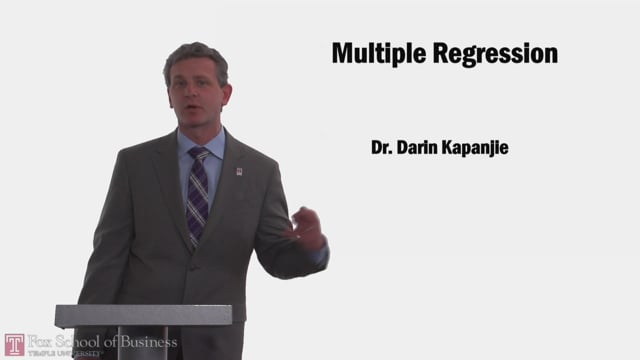Multiple Regression
Total Running Time: 12:32
Multiple Regression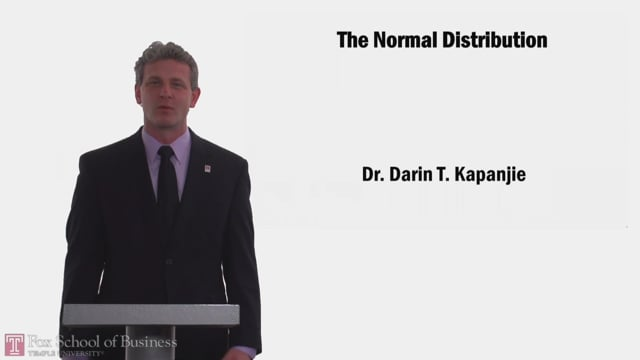Normal Distribution
Total Running Time: 16:42
Normal Distribution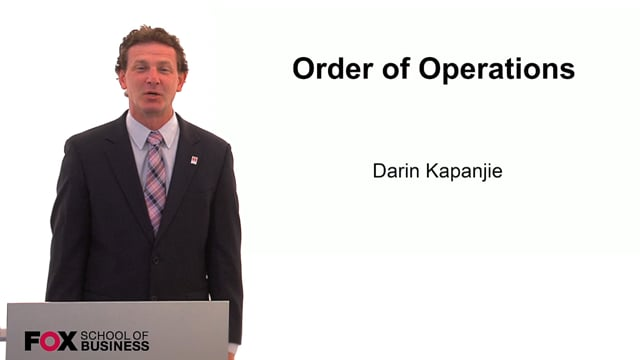Order of Operations
Total Running Time: 08:13
Order of OperationsPolynomials
Total Running Time: 17:14
PolynomialsProportions
Total Running Time: 04:32
ProportionsTotal Running Time: 31:25Total Running Time: 22:26Rational Expressions
Total Running Time: 14:10
Rational ExpressionsRational Expressions
Total Running Time: 14:10
Rational Expressions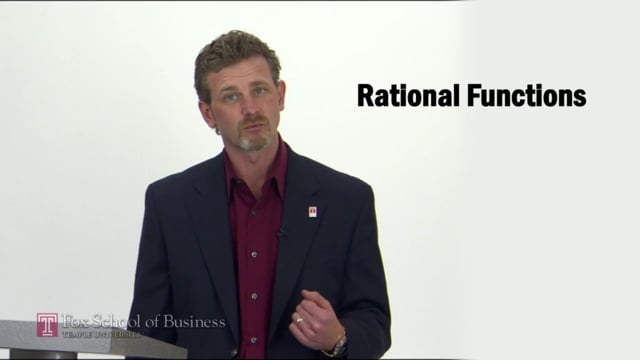Rational Functions
Total Running Time: 15:11
Rational FunctionsRatios
Total Running Time: 03:10
Ratios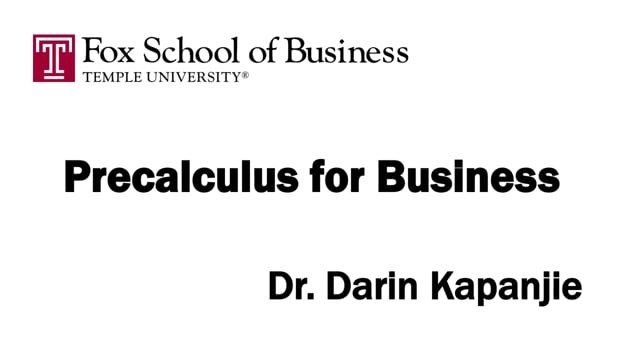Real Numbers
Total Running Time: 16:50
Real NumbersSampling Distribution
Total Running Time: 22:29
Sampling Distribution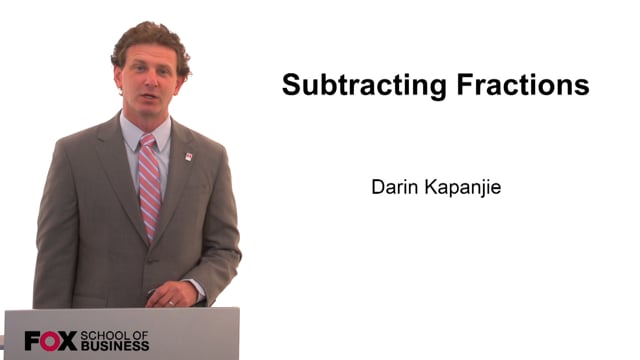Subtracting Fractions
Total Running Time: 06:19
Subtracting FractionsSurveys & Sampling
Total Running Time: 18:58
Surveys & SamplingTesting Hypotheses
Total Running Time: 22:52
Testing HypothesesGo Back to the top of the pageFox Video Vault vault.temple.edu